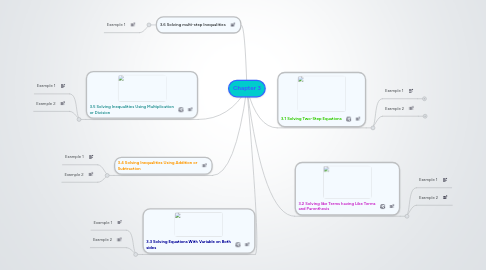# Chapter 3

AB
A B
Get Started. It's FreeChapter 3 by## 1. 3.1 Solving Two-Step Equations

### 1.1. Example 1

1.1.1. << using subtraction and division to solve .

### 1.2. Example 2

1.2.1. << using addition and multiplication to solve.